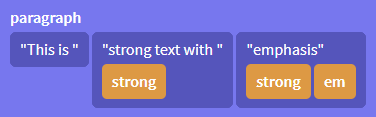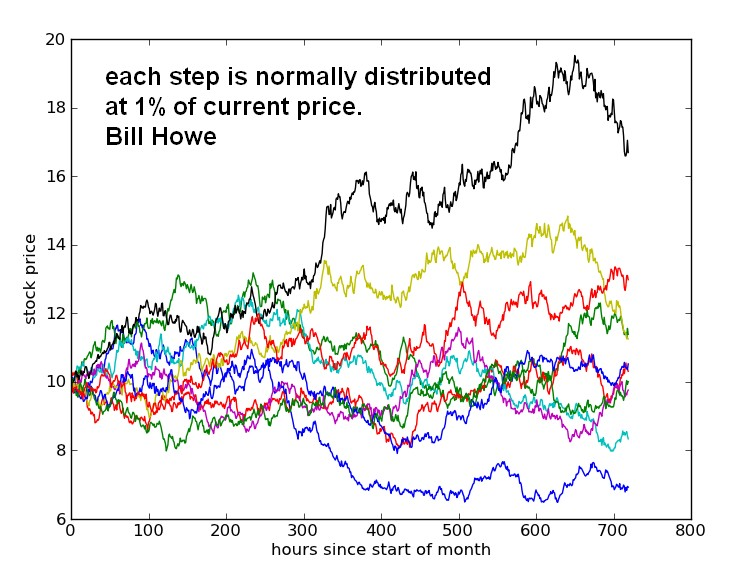# HTML - Canvas Element: Example

Note before going on the examples: On a canvas drawing surface, the 2D Canvas Context is the API and provides:

• objects,
• methods,
• and properties to draw and manipulate graphics.

## Example

### Drawing the origin

function graphAxes(width, height) {

ctx.beginPath();
ctx.strokeStyle = "black";
// X axis
ctx.moveTo(0, 0);
ctx.lineTo(width, 0);
// Y axis
ctx.moveTo(0, 0);
ctx.lineTo(0,height);
ctx.stroke();
}

// Main Global Scope
canvas = document.getElementById("graph");
ctx = canvas.getContext("2d");
graphAxes(600,300);

<canvas id="graph" width="600" height="300"></canvas>

• Output: the 2 axis

### How to create a Function Grapher

function graphFunction(graph, func, color) {

// A point every 4 pixels
var dx = 4;

// Properties
ctx.strokeStyle = color;

// Begin Path Graphing
ctx.beginPath();
for (var x = graph.xMin; x <= graph.xMax; x = x+dx) {
y = graph.yScale * func(x / graph.xScale);
if (x == graph.xMin) {
ctx.moveTo(graph.x0 + x, graph.y0 - y);
}
else ctx.lineTo(graph.x0 + x, graph.y0 - y);
}
ctx.stroke();
}

function graphAxes(graph) {

ctx.beginPath();
ctx.strokeStyle = "black";
// X axis
ctx.moveTo(0, graph.y0);
ctx.lineTo(graph.width, graph.y0);
// Y axis
ctx.moveTo(graph.x0, 0);
ctx.lineTo(graph.x0, graph.height);
ctx.stroke();
}

// Main Global Scope
canvas = document.getElementById("graph");
ctx = canvas.getContext("2d");

// Graph Properties
var graph = {
x0: .5 + .5 * canvas.width,  // x0 pixels from left to x=0
y0: .5 + .5 * canvas.height, // y0 pixels from top to y=0
get xMax(){ return canvas.width - this.x0},
get xMin(){ return - this.x0},
xScale: 40,                 // Number of pixels from x=0 to x=1
yScale: 100,                 // Number of pixels from y=0 to y=1
width: canvas.width,
height: canvas.height
};

// Graph

// The axes
graphAxes(graph);

// The first Function
var sin = (x) => Math.sin(x);
graphFunction(graph, sin, "blue");

// The second Function
var cos = (x) => Math.cos(x);
graphFunction(graph, cos, "red");

<canvas id="graph" width="600" height="300"></canvas>


let download = function(selector){
let canva = document.querySelector(selector);
}


## Export format

Browsers canvas implementation only generate raster images, and don't help with SVG or PDF. https://github.com/bokeh/bokeh/issues/538#issuecomment-157931426

## Documentation / Specification

Discover MoreData Visualization - Graphic (Tool|Software|Api|Library)

Flexibility Usability Sofware / Tool 0 3 Excel, GUI 1 2 Declarative (Dimple.js based on d3) 2 1 Imperative (D3.js) 3 0 Canvas, WebGL, SVG Graphics in the browser:...
HTML - (Flow|Body) Content

Most elements that are used in the body of documents and applications are categorized as flow content. Flow content consists of flow elements intermixed with normal character data. (ifbodhead...
HTML - Embedded Content

Embedded content is content that imports another resource into the document, or content from another vocabulary that is inserted into the document. HTML Elements that...
HTML - Palpable content

As a general rule, elements whose content model allows any flow content or phrasing content should have at least one node in its contents: that is palpable content (Can be manipulated ?) and that...
HTML - Phrasing Content (Text)

Phrasing content is: the text of the document, as well as elements that mark up that text at the intra-paragraph level. Runs of phrasing content form paragraphs. Most elements that are categorized...HTML - WebGL (3D rendering)

WebGL™ is an immediate mode 3D rendering API designed for the web. It is derived from OpenGL® ES 2.0, and provides similar rendering functionality, but in anHTML context. WebGL is built on top of the...How Rich Text editor in HTML are made (Principles and Demo)

How do you create a Rich Text editor in HTML, what are the well-known text editor and what are the principals. This article includes also a basic example where you can extend from to build your ownScalable Vector Graphics (SVG)

SVG is a markup language describing two-dimensional image in XML . You just have to declare the visuals that you want. SVG allows for three types of graphic objects: vector graphic shapes (e.g.,...Statistics - Random Walk

A random walk is a mathematical formalization of a path that consists of a succession of random steps. For example, the price of a fluctuating stock can be modelled as random walks. Half the time it goes...